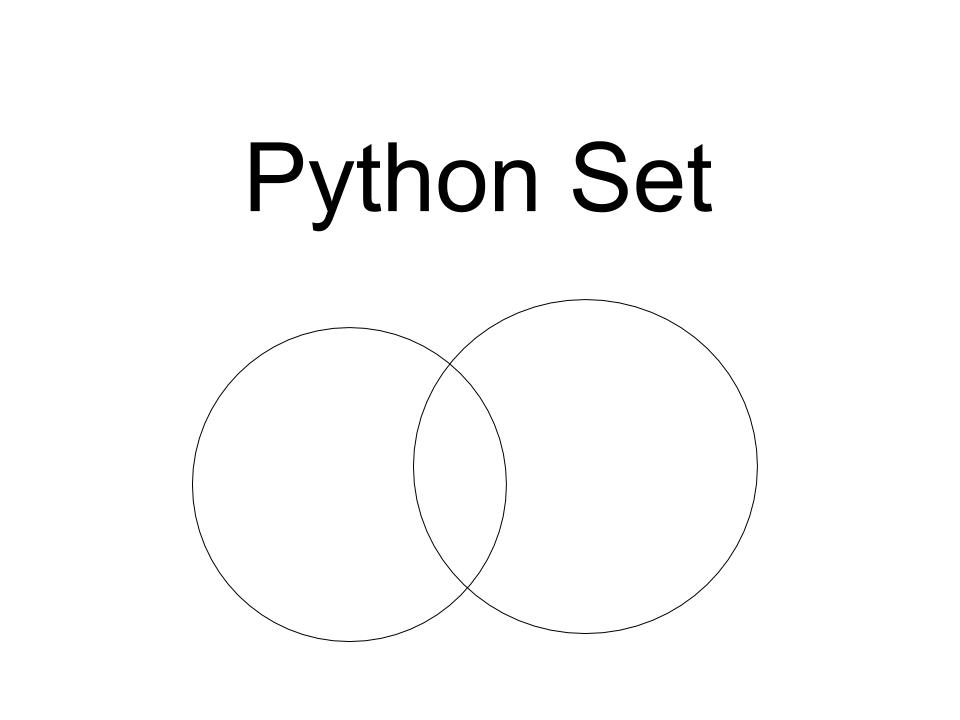# Python Set

Published on August 3, 2022By Pankaj
Developer and author at DigitalOcean.While we believe that this content benefits our community, we have not yet thoroughly reviewed it. If you have any suggestions for improvements, please let us know by clicking the “report an issue“ button at the bottom of the tutorial.

In this tutorial we are going to learn Python Set. In our previous article we learnt about Python String. You can learn it from here.## Python Set

Python Set is an unordered collection of unique elements. Suppose you have a list and you need only the unique items of the list you can use Python Set. Similarly, if you need only unique items from input, Python set can help you to do so. You can add or delete items from it. You can initialize a set by placing elements in between curly braces. Like other sequences, one set can have elements of multiple data-types. Moreover, you can also create a set from a list by using set() function. The following example will give you some idea about initializing a set.

``````#set containing single data-type
set1 = {1, 2, 3, 4, 2, 3, 1}
print(set1)

#set containing multiple data-type
set2 = {1, 2, 3, (1, 2, 3), 2.45, "Python", 2, 3}
print(set2)

#creating a set from a list
theList = [1, 2, 3, 4, 2, 3, 1]
theSet = set(theList)
print(theSet)
``````

The output will be

``````================== RESTART: /home/imtiaz/set1.py ==================
set([1, 2, 3, 4])
set([1, 2, 3, 2.45, 'Python', (1, 2, 3)])
set([1, 2, 3, 4])
>>>
``````

### Adding Elements to Python Set

In previous example, we learned how to initialize Python set directly. Suppose we need to add element to set, we can do so by using add() function. But this function can add a single element. If you want to add iterable elements like list or set, you can do so by using update() function. The following example will help you understand the thing

``````#initialize an empty set
theSet = set()

#add iterable elements using update() function
theSet.update([1,2,4,'hello','world']) #list as iterable element
theSet.update({1,2,5}) #set as iterable element
print(theSet)
``````

The output of the following code will be

``````================== RESTART: /home/imtiaz/set_new.py ==================
set([1, 2, 3, 4, 5, 'world', 'hello'])
>>>
``````

### Remove Elements from Python Set

There are two functions to remove elements from Python Set. One is remove() and another is discard() function. If the element you are trying to remove is not in the set, the remove() function will raise exception for this. But the discard function will not do anything like this. The following code will show you those

``````theSet = {1,2,3,4,5,6}

print(theSet)

#call discard() function again to remove 3
theSet.discard(3) #This won't raise any exception
print(theSet)

#call remove() function to remove 5
theSet.remove(5)
print(theSet)

#call remove() function to remove 5 again
theSet.remove(5) #this would raise exception
print(theSet) #this won't be printed
``````

You will find the output be like,

``````================== RESTART: /home/imtiaz/set_del.py ==================
set([1, 2, 4, 5, 6])
set([1, 2, 4, 5, 6])
set([1, 2, 4, 6])

Traceback (most recent call last):
File "/home/imtiaz/set_del.py", line 16, in
theSet.remove(5) #this would raise exception
KeyError: 5
>>>
``````

### Python Set Operations

You might be familiar with some mathematical set operations like union, intersection, difference. We can also do those using Python set. Now, we will learn how to do that.

### Python Set Union

Union is the operation to merge two sets. That means, union will create another set that contains all unique elements of two sets. For example, {1, 2, 3, 4} and {2, 3, 5, 7} are two sets. If we do union operation over them, we get {1, 2, 3, 4, 5, 7}. We can obtain this by using union() function.

### Python Set Intersection

Again, intersection is the operation to get the common unique elements of two sets. For example, {1, 2, 3, 4} and { 2, 3, 5, 7} are two sets. If we intersect them, we get, {2, 3}. The intersection operation is done by intersection() function.

### Python Set Difference

Now, difference operation compares two sets and creates a new set containing items from set A which are not common in set B. Suppose, we have two sets, A = {1, 2, 3, 4} and B = {2, 3, 5, 7}. Then, A - B operation will generate {1, 4}. Moreover, B - A will generate {5, 7}. The difference operation is done by difference() function… The following code will give you idea about how to do these set operation in python programming.

``````A = {1, 2, 3, 4} #initializing set A
B = {2, 3, 5, 7} #initializing set B

union_operation = A.union(B)

print("A union B :")
print(union_operation)

intersection_operation = A.intersection(B)

print("A intersection B :")
print(intersection_operation)

difference_operation = A.difference(B)

print("A-B :")
print(difference_operation)

difference_operation = B.difference(A)
print("B-A :")
print(difference_operation)
``````

The output you get will be like this

``````================== RESTART: /home/imtiaz/set_op.py ==================
A union B :
set([1, 2, 3, 4, 5, 7])
A intersection B :
set([2, 3])
A-B :
set([1, 4])
B-A :
set([5, 7])
>>>
``````

So, that’s all for today. Hope that you learned well about Python Set. For any further query you can just write your query in the comment box. We will answer you. Reference: Official DocumentationPankaj

author

Developer and author at DigitalOcean.